# GSEB Solutions Class 12 Maths Chapter 9 Differential Equations Ex 9.4

Gujarat Board GSEB Textbook Solutions Class 12 Maths Chapter 9 Differential Equations Ex 9.4 Textbook Questions and Answers.

## Gujarat Board Textbook Solutions Class 12 Maths Chapter 9 Differential Equations Ex 9.4

For each of the differential equations in questions 1 to 10, find the general solution:
Question 1.
$$\frac{dy}{dx}$$ = $$\frac{1-cosx}{1+cosx}$$
Solution: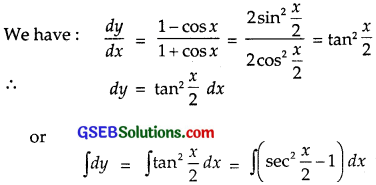∴ y = 2 tan $$\frac{x}{2}$$ – x + C
This is the required solution.Question 2.
$$\frac{dy}{dx}$$ = $$\sqrt{4-y^{2}}$$ (- 2 < y < 2)
Solution:
$$\frac{dy}{dx}$$ = $$\sqrt{4-y^{2}}$$ or $$\frac{d y}{\sqrt{4-y^{2}}}$$ = dx
Integrating both sides, we get
∫ $$\frac{d y}{\sqrt{4-y^{2}}}$$ = ∫dx
⇒ sin-1$$\frac{y}{2}$$ = x + C or y = 2 sin(x + C).
which is the required solution.

Question 3.
$$\frac{dy}{dx}$$ + y = 1 (y ≠ 1)
Solution:
Given differential equation is $$\frac{dy}{dx}$$ + y = 1
or $$\frac{dy}{dx}$$ = 1 – y or $$\frac{dy}{1-y}$$ = dx
Integrating both sides, we get
∫ – log (1 – y) = x + log C
∴ x = – log C – log (1 – y) = – log C(1 – y)
∴ C(1 – y) = e-x
or C(1 – y)ex = 1
⇒ 1 – y = $$\frac{1}{C}$$e-x ⇒ y = 1 – $$\frac{1}{C}$$e-x.
Put – $$\frac{1}{C}$$ = A; y = 1 + Ae-x is the required solution.Question 4.
sec2x tan y dx + sec2y tan x dy = 0
Solution:
We have:
sec2x tan y dx + sec2y tan x dy = 0
⇒ $$\frac{\sec ^{2} x}{\tan x}$$ dx + $$\frac{\sec ^{2} x}{\tan y}$$dy = 0
Integrating both sides, we get
∫ $$\frac{\sec ^{2} x}{\tan x}$$ dx + ∫ $$\frac{\sec ^{2} y}{\tan y}$$dy = 0
⇒ log|tan x| + log|tan y| = log C
⇒ log|tan x tan y| = log C
⇒ tan x tan y = C
which is the required solution, where x ≠ odd multiple of and x ∈ R.

Question 5.
(ex + e-x)dy – (ex – e-x)dx = 0
Solution:
We have:
(ex + e-x)dy = (ex – e-x)dx
⇒ dy = $$\frac{e^{x}-e^{-x}}{e^{x}+e^{-x}}$$dx
Integrating both sides, we get
∫ dy = ∫ $$\frac{e^{x}-e^{-x}}{e^{x}+e^{-x}}$$dx
⇒ ∫ dy = ∫$$\frac{dt}{t}$$,
where ex + e-x = t so that (ex – e-x) dx = dt.
⇒ y = log |t| + C
⇒ y – C = log|ex + e-x| (x ∈ R)
or y = log (ex + e-x) + C (∵ ex, e-x > 0)
which is the required solution.Question 6.
$$\frac{dy}{dx}$$ = (1 + x)2(1 + y2)
Solution:
$$\frac{dy}{dx}$$ = (1 + x)2(1 + y2)
or $$\frac{d y}{1+y^{2}}$$ = (1 + x2) dx
Integrating both sides, we get
∫ $$\frac{d y}{1+y^{2}}$$ = ∫
⇒ tan-1y = x + $$\frac{x^{3}}{3}$$ + C,
which is the required equation.

Question 7.
y log y dx – x dy = 0
Solution:
y log y dx – x dy = 0
or y log y dx = x dy or $$\frac{dy}{ylogy}$$ = $$\frac{dx}{x}$$
Integrating both sides, we get
∫ $$\frac{dy}{ylogy}$$ = ∫$$\frac{dx}{x}$$
Putting log y = t, $$\frac{1}{y}$$dy = dt
So, ∫ $$\frac{dt}{t}$$ = log x + log C
∴ log(log y) = log Cx
∴ log y = Cx
or y = eCx is the required Solution.Question 8.
x5$$\frac{dy}{dx}$$ = – y5
Solution: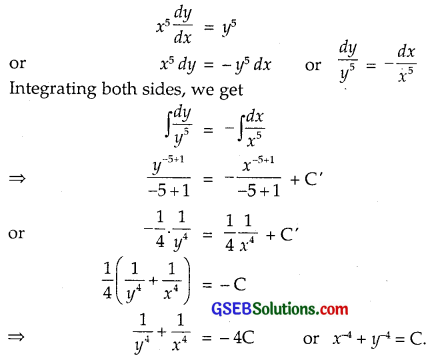Putting – 4C = C. This is the required solution.

Question 9.
$$\frac{dy}{dx}$$ = sin-1x
Solution:
$$\frac{dy}{dx}$$ = sin-1x
or dy = sin-1x
Integrating both sides, we get
∫ dy = ∫sin-1 x dx + C
or y = ∫(sin-1 x). 1 dx + C
Integrating by parts, taking sin-1x as first function, we get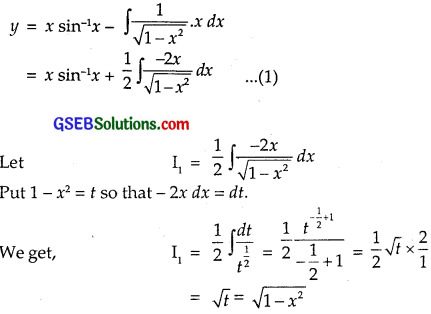Putting this value of I1 in (1), we get
y = x sin-1x + $$\sqrt{1-x^{2}}$$ + C
∴ The Solution is y = x sin-1x + $$\sqrt{1-x^{2}}$$ + C.Question 10.
extan y dx + (1 – ex) sec2y dy = 0
Solution:
ex tan y dx + (1 – ex) sec2y dy = – ex tan y dx = 0
or (1 – ex) sec2y dy = – ex tan y dx
Dividing by (1 – ex) tan, we get
$$\frac{\sec ^{2} y}{\tan y}$$ dy = + $$\frac{-e^{x}}{1-e^{x}}$$dx
Integrating both sides, we get
∫ $$\frac{\sec ^{2} y}{\tan y}$$dy = ∫$$\frac{-e^{x}}{1-e^{x}}$$dx
∴ log tan y = log (1 – e-x) + log C
= log C(1 – e-x)
∴ tan y = C(1 – e-x) is the required solution.

For each of the differential equations in questions from 11 to 14, find a particular solution satisfying the given condition:

Question 11.
(x3 + x2 + x + 1)$$\frac{dy}{dx}$$ = 2x2 + x; y = 1, when x = 0
Solution:
(x3 + x2 + x + 1)$$\frac{dy}{dx}$$ = 2x2 + x
or (x3 + x2 + x + 1)dy = (2x2 + x)dx
or dy = $$\frac{2 x^{2}+x}{x^{3}+x^{2}+x+1}$$ dx
Integrating both sides, we get⇒ 2x2 + x = A(x2 + 1) + (Bx + C)(x + 1)
= A(x2 + 1) + B(x2 + x) + C(x + 1) ………….. (2)
Put x = – 1 in (2), we get
2 – 1 = A(1 + 1) ⇒ A = $$\frac{1}{2}$$
Comparing the coefficients of x2 and x, we get
2 = A + B and 1 = B + C.
∴ B = 2 – A = 2 – $$\frac{1}{2}$$ = $$\frac{3}{2}$$.
C = 1 – B = 1 – $$\frac{3}{2}$$ = – $$\frac{1}{2}$$.Therefore, from (1), we getWe have y = 1, when x = 0.
Putting these values in (3), we get
1 = $$\frac{1}{2}$$ log 1 + $$\frac{3}{4}$$ log 1 – $$\frac{1}{2}$$tan-10 + C ……………. (3)
[Note : for integrating ∫ $$\frac{2 x}{x^{2}+1}$$dx, put x2 + 1 = t
We have y = 1, when x = 0.
Putting these values in (3), we get
1 = $$\frac{1}{2}$$ log 1 + $$\frac{3}{4}$$ log 1 – $$\frac{1}{2}$$tan-10 + C
= 0 + C ⇒ C = 1.
Thus, the solution is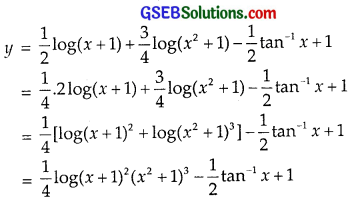This is the required particular solution.Question 12.
x(x2 – 1)$$\frac{dy}{dx}$$ = 1; y = 0, when x = 2
Solution:Question 13.
cos($$\frac{dy}{dx}$$) = a; (a ∈ R), y = 2, when x = 0
Solution: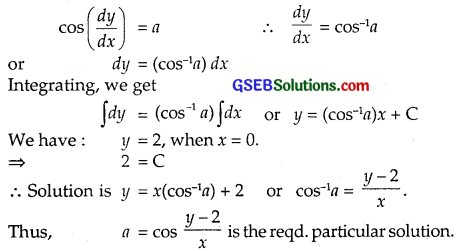Question 14.
$$\frac{dy}{dx}$$ = y tan x; y = 1, when x = 0
Solution:
$$\frac{dy}{dx}$$ = y tan x or $$\frac{dy}{y}$$ = tan x dx
Integrating, we get
∫ $$\frac{dy}{y}$$ = ∫ tan x dx
⇒ log y = – log cos x + log C
or log y + log cos x = log C
∴ y cos x = C
Putting y = 1, x = 0 and ⇒ C = 1.
∴ y cos x = C
Putting y = 1, x = 0 ⇒ C = 1.
∴ y cos x = 1
or y = sec x is the required particular solution.Question 15.
Find the equation of the curve passing through the point (0, 0) and whose differential equation is y’ = ex sin x.
Solution:
y’ = exsin x or $$\frac{dy}{dx}$$ = exsin x
∴ dy = ex sin x dx
Integrating both sides, we get
∫ dy = ∫ex sin x dx
Integrating by parts, taking ex as the first function, we get
y = ex(- cos x) – ∫ex sin x dx
= – excos x + ∫excos x dx
Again integrating by parts, taking ex as first function, we get
y = – ex cos x + ex sin x – ∫ex sin x dx
⇒ y = – ex cos x + ex sin x – ∫dy
⇒ y = – ex cos x + ex sin x + C
∴ y = $$\frac{e^{x}}{2}$$[- cos x + sin x] + C
Put x = 0, y = 0.
0 = – $$\frac{1}{2}$$ + C ∴ C = $$\frac{1}{2}$$.
∴ Solution is y = $$\frac{e^{x}}{2}$$(sin x – cos x) + $$\frac{1}{2}$$
Which is the required equation of the curve.

Question 16.
If for the differential equation xy$$\frac{dy}{dx}$$ = (x + 2)(y + 2), find the solution curve passing through the point (1, – 1).
Solution:
The differential equation is xy$$\frac{dy}{dx}$$ = (x + 2)(y + 2)
or xy dy = (x + 2)(y + 2)dx
Dividing by x(y + 2), we get
$$\frac{y}{y+2}$$dy = $$\frac{x+2}{x}$$dx
Integrating, we get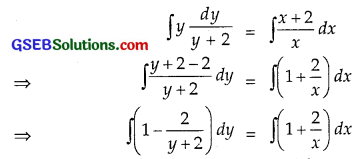⇒ y – 2 log (y + 2) = x + 2 log x + C
The curve passes through (1, – 1)
∴ – 1 – 2 log 1 = 1 + 2 log 1 + C
⇒ – 1 = 1 + C [log 1 = 0]
∴ C = – 2.
Putting C = – 2, we get
y – 2 log (y + 2) = x + 2 log x – 2
or y – x = 2[log (y + 2) + log x] – 2
= 2 log x (y + 2) – 2
∴ Solution curve is y = x + 2 log x (y + 2) – 2.Question 17.
Find the equation of the curve passing through the point (0, – 2) given that at any point (x, y) on the curve,
the product of the slope of its tangent and y coordinate of the point is equal to the x coordinate of the point.
Solution:
Slope of tangent to the curve at (x, y) = $$\frac{dy}{dx}$$.
We are given: y($$\frac{dy}{dx}$$) = x
∴ ydy = xdx
Integrating, we get
$$\frac{y^{2}}{2}$$ = $$\frac{x^{2}}{2}$$ + C
or y2 = x2 + 2C.
The curve passes through (0, – 2).
∴ 4 = 0 + 2C.
∴ 2C = 4.
∴ Equation of the curve is
y2 = x2 + 4 or y2 – x2 = 4.

Question 18.
At any point (x, y) of a curve, the slope of the tangent is twice the slope of the line segment joining the point of contact to the point (- 4, – 3).
Find the equation of the curve given that it passes through (- 2, 1).
Solution:
Slope of the tangent to the curve = $$\frac{dy}{dx}$$
Slope of the line joining (x, y) and (- 4, – 3) = $$\frac{y+3}{x+4}$$
We are given: $$\frac{dy}{dx}$$ = 2($$\frac{y+3}{x+4}$$)
∴ dy = $$\frac{2(y+3)}{x+4}$$ dx
Dividing by y + 3, we get
∴ $$\frac{dy}{y+3}$$ = $$\frac{2}{x+4}$$dx
Integrating, we get
∫ $$\frac{dy}{y+3}$$ = 2∫$$\frac{dx}{x+4}$$
or log(y + 3) = 2 log (x + 4) + log C
or log (y + 3) – log (x + 4)2 = log C
i.e; log $$\frac{y+3}{(x+4)^{2}}$$ = C ∴ $$\frac{y+3}{(x+4)^{2}}$$ = C.
The curve passes through (- 2, 1).
∴ $$\frac{1+3}{(-2+4)^{2}}$$ = C = $$\frac{4}{4}$$ = 1.
∴ Equation of the curve is $$\frac{y+3}{(x+4)^{2}}$$ = 1.
or y + 3 = (x + 4)2 or y = (x + 4)2 – 3.Question 19.
The volume of a spherical balloon, being inIated, changes at a constant rate.
If initially its radius is 3 units and after 3 seconds it is 6 units, find the radius of balloon after t seconds.
Solution:
Let V be the volume of the balloon.Putting values of k and C in (1), we get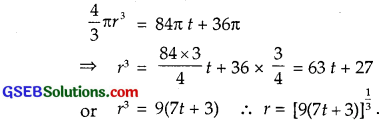Question 20.
In a bank, principal increases at the rate of r% per year. Find the value of r, if ₹100 double itself in 10 years (log 2 = 0.6931).
Solution:
Let p be the principal an rate of interest = r%.∴ r = 10 × 0.6931 = 6.931.
Thus, the value of r = 6.931.

Question 21.
In a bank, principal increases at the rate of 5% per year.
An amount of ₹1000 is deposited with this bank. How much will it worth after 10 years (e0.5 = 1.648)?
Solution:
Let p be the principal and Rate of interest is 5%.
∴ $$\frac{dp}{dt}$$ = $$\frac{5}{100}$$p
∴ $$\frac{dp}{p}$$ = 0.05 dt
Integrating, we get log p = 0.05 t + log C
or log p – log C = 0.05 t or log $$\frac{p}{C}$$ = 0.05 t.
$$\frac{p}{C}$$ = e0.005t ∴ p = Ce0.005t …………… (1)
Initially p = ₹1000, t = 0
∴ 1000 = C e0 = C
∴ C = 1000.
Putting this value in (1), we get
p = 1000 e0.005t
When t = 10, p = 1000 e0.005×10 = 1000 e0.5
p = 1000 × 1.648 [∵ e0.5 = 1.648]
= 1648
After 10 years, ₹1000 will amount to ₹1648.Question 22.
In a culture, the bacteria count is 1,00,000. The number is increased by 10% in 2 hours.
In how many hours, will the count reach 2,00,000, if the rate of growth of bacteria is proportional to the number present?
Solution:
Let y denote the number of bacteria at any instant t.
Then, according to the problem,
$$\frac{dy}{dt}$$ ∝ y
⇒ $$\frac{dy}{y}$$ = k dt ……………… (1)
where k is the constant of proportionality, taken to be positive. On integrating (1), we get
log y = kt + C ………………. (2)
where C is a parameter.
Let y0 be the initial number of bacteria, i.e., at t = 0.
Using this in (2), we get C = log y0.
So, we have: log y = kt + log y0
⇒ log $$\frac{y}{y_{0}}$$ = kt
According to the problem,
y = (y0 + $$\frac{10}{100}$$y0) = $$\frac{11 y_{0}}{10}$$, when t = 2
So, from (3) we get,Le the number of bacteria becomes from 1,00,000 to 2,00,000 in t1 hours i.e; y = 2y0, when t = t1 hours.
Using (5) we get,Question 23.
The general solution of the differential equation $$\frac{dy}{dx}$$ = ex+y is
(A) ex + e-y = c
(B) ex + ey = c
(C) e-x + ey = c
(D) e-x + e-y = c
Solution:
$$\frac{dy}{dx}$$ = ex+y = ex.ey
dy = ex.ey dx
Dividing by ey, we get
e-ydy = exdx
Integrating, we get
∫ e-y dy = ∫ ex dx
or – e-y = e-x – c = ex + e-y = c.
∴ Part (A) is the correct answer.